## Access Answers of Maths NCERT Class 9 Maths Chapter 6 – Lines And Angles

### Class 9 Maths Chapter 6 Exercise: 6.1 (Page No: 96)

Exercise: 6.1 (Page No: 96)

1. In Fig. 6.13, lines AB and CD intersect at O. If ∠AOC +∠BOE = 70° and ∠BOD = 40°, find ∠BOE and reflex ∠COE.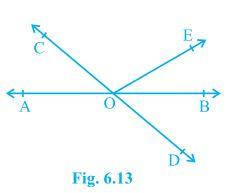Solution:

From the diagram, we have

(∠AOC +∠BOE +∠COE) and (∠COE +∠BOD +∠BOE) forms a straight line.

So, ∠AOC+∠BOE +∠COE = ∠COE +∠BOD+∠BOE = 180°

Now, by putting the values of ∠AOC + ∠BOE = 70° and ∠BOD = 40° we get

∠COE = 110° and ∠BOE = 30°

So, reflex ∠COE = 360o – 110o = 250o

2. In Fig. 6.14, lines XY and MN intersect at O. If ∠POY = 90° and a : b = 2 : 3, find c.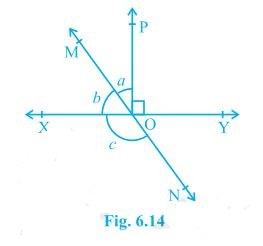Solution:

We know that the sum of linear pair are always equal to 180°

So,

∠POY +a +b = 180°

Putting the value of ∠POY = 90° (as given in the question) we get,

a+b = 90°

Now, it is given that a : b = 2 : 3 so,

Let a be 2x and b be 3x

∴ 2x+3x = 90°

Solving this we get

5x = 90°

So, x = 18°

∴ a = 2×18° = 36°

Similarly, b can be calculated and the value will be

b = 3×18° = 54°

From the diagram, b+c also forms a straight angle so,

b+c = 180°

c+54° = 180°

∴ c = 126°

3. In Fig. 6.15, ∠PQR = ∠PRQ, then prove that ∠PQS = ∠PRT.Solution:

Since ST is a straight line so,

PQS+PQR = 180° (linear pair) and

PRT+PRQ = 180° (linear pair)

Now, PQS + PQR = PRT+PRQ = 180°

Since PQR =PRQ (as given in the question)

PQS = PRT. (Hence proved).

4. In Fig. 6.16, if x+y = w+z, then prove that AOB is a line.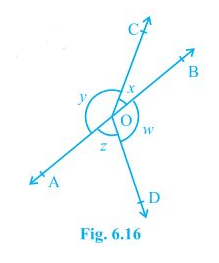Solution:

For proving AOB is a straight line, we will have to prove x+y is a linear pair

i.e. x+y = 180°

We know that the angles around a point are 360° so,

x+y+w+z = 360°

In the question, it is given that,

x+y = w+z

So, (x+y)+(x+y) = 360°

2(x+y) = 360°

∴ (x+y) = 180° (Hence proved).

5. In Fig. 6.17, POQ is a line. Ray OR is perpendicular to line PQ. OS is another ray lying between rays OP and OR. Prove that ∠ROS = ½ (∠QOS – ∠POS).Solution:

In the question, it is given that (OR ⊥ PQ) and ∠POQ = 180°

So, ∠POS+∠ROS+∠ROQ = 180°

Now, ∠POS+∠ROS = 180°- 90° (Since ∠POR = ∠ROQ = 90°)

∴ ∠POS + ∠ROS = 90°

Now, ∠QOS = ∠ROQ+∠ROS

It is given that ∠ROQ = 90°,

∴ ∠QOS = 90° +∠ROS

Or, ∠QOS – ∠ROS = 90°

As ∠POS + ∠ROS = 90° and ∠QOS – ∠ROS = 90°, we get

∠POS + ∠ROS = ∠QOS – ∠ROS

2 ∠ROS + ∠POS = ∠QOS

Or, ∠ROS = ½ (∠QOS – ∠POS) (Hence proved).

6. It is given that ∠XYZ = 64° and XY is produced to point P. Draw a figure from the given information. If ray YQ bisects ∠ZYP, find ∠XYQ and reflex ∠QYP.

Solution: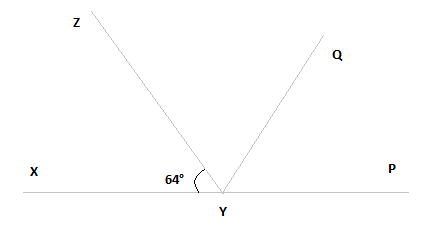Here, XP is a straight line

So, ∠XYZ +∠ZYP = 180°

Putting the value of ∠XYZ = 64° we get,

64° +∠ZYP = 180°

∴ ∠ZYP = 116°

From the diagram, we also know that ∠ZYP = ∠ZYQ + ∠QYP

Now, as YQ bisects ∠ZYP,

∠ZYQ = ∠QYP

Or, ∠ZYP = 2∠ZYQ

∴ ∠ZYQ = ∠QYP = 58°

Again, ∠XYQ = ∠XYZ + ∠ZYQ

By putting the value of ∠XYZ = 64° and ∠ZYQ = 58° we get.

∠XYQ = 64°+58°

Or, ∠XYQ = 122°

Now, reflex ∠QYP = 180°+XYQ

We computed that the value of ∠XYQ = 122°.

So,

∠QYP = 180°+122°

∴ ∠QYP = 302°

Exercise: 6.2 (Page No: 103)

1. In Fig. 6.28, find the values of x and y and then show that AB || CD.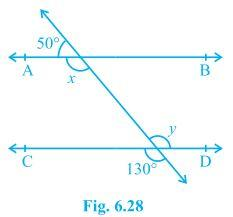Solution:

We know that a linear pair is equal to 180°.

So, x+50° = 180°

∴ x = 130°

We also know that vertically opposite angles are equal.

So, y = 130°

In two parallel lines, the alternate interior angles are equal. In this,

x = y = 130°

This proves that alternate interior angles are equal and so, AB || CD.

2. In Fig. 6.29, if AB || CD, CD || EF and y : z = 3 : 7, find x.Solution:

It is known that AB || CD and CD||EF

As the angles on the same side of a transversal line sums up to 180°,

x + y = 180° —–(i)

Also,

∠O = z (Since they are corresponding angles)

and, y +∠O = 180° (Since they are a linear pair)

So, y+z = 180°

Now, let y = 3w and hence, z = 7w (As y : z = 3 : 7)

∴ 3w+7w = 180°

Or, 10 w = 180°

So, w = 18°

Now, y = 3×18° = 54°

and, z = 7×18° = 126°

Now, angle x can be calculated from equation (i)

x+y = 180°

Or, x+54° = 180°

∴ x = 126°

3. In Fig. 6.30, if AB || CD, EF ⊥ CD and ∠GED = 126°, find ∠AGE, ∠GEF and ∠FGE.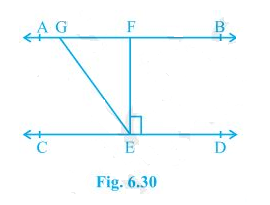Solution:

Since AB || CD, GE is a transversal.

It is given that ∠GED = 126°

So, ∠GED = ∠AGE = 126° (As they are alternate interior angles)

Also,

∠GED = ∠GEF +∠FED

As EF⊥ CD, ∠FED = 90°

∴ ∠GED = ∠GEF+90°

Or, ∠GEF = 126° – 90° = 36°

Again, ∠FGE +∠GED = 180° (Transversal)

Putting the value of ∠GED = 126° we get,

∠FGE = 54°

So,

∠AGE = 126°

∠GEF = 36° and

∠FGE = 54°

4. In Fig. 6.31, if PQ || ST, ∠PQR = 110° and ∠RST = 130°, find ∠QRS.

[Hint : Draw a line parallel to ST through point R.]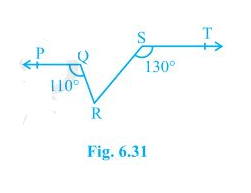Solution:

First, construct a line XY parallel to PQ.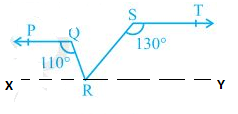We know that the angles on the same side of transversal is equal to 180°.

So, ∠PQR+∠QRX = 180°

Or, ∠QRX = 180°-110°

∴ ∠QRX = 70°

Similarly,

∠RST +∠SRY = 180°

Or, ∠SRY = 180°- 130°

∴ ∠SRY = 50°

Now, for the linear pairs on the line XY-

∠QRX+∠QRS+∠SRY = 180°

Putting their respective values, we get,

∠QRS = 180° – 70° – 50°

Hence, ∠QRS = 60°

5. In Fig. 6.32, if AB || CD, ∠APQ = 50° and ∠PRD = 127°, find x and y.Solution:

From the diagram,

∠APQ = ∠PQR (Alternate interior angles)

Now, putting the value of ∠APQ = 50° and ∠PQR = x we get,

x = 50°

Also,

∠APR = ∠PRD (Alternate interior angles)

Or, ∠APR = 127° (As it is given that ∠PRD = 127°)

We know that

∠APR = ∠APQ+∠QPR

Now, putting values of ∠QPR = y and ∠APR = 127° we get,

127° = 50°+ y

Or, y = 77°

Thus, the values of x and y are calculated as:

x = 50° and y = 77°

6. In Fig. 6.33, PQ and RS are two mirrors placed parallel to each other. An incident ray AB strikes the mirror PQ at B, the reflected ray moves along the path BC and strikes the mirror RS at C and again reflects back along CD. Prove that AB || CD.Solution:

First, draw two lines BE and CF such that BE ⊥ PQ and CF ⊥ RS.

Now, since PQ || RS,

So, BE || CF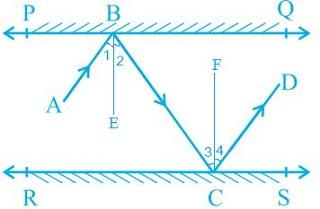We know that,

Angle of incidence = Angle of reflection (By the law of reflection)

So,

∠1 = ∠2 and

∠3 = ∠4

We also know that alternate interior angles are equal. Here, BE ⊥ CF and the transversal line BC cuts them at B and C

So, ∠2 = ∠3 (As they are alternate interior angles)

Now, ∠1 +∠2 = ∠3 +∠4

Or, ∠ABC = ∠DCB

So, AB || CD (alternate interior angles are equal)

Exercise: 6.3 (Page No: 107)

1. In Fig. 6.39, sides QP and RQ of ΔPQR are produced to points S and T respectively. If ∠SPR = 135° and ∠PQT = 110°, find ∠PRQ.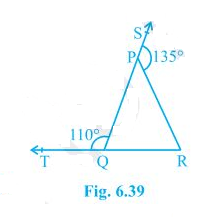Solution:

It is given the TQR is a straight line and so, the linear pairs (i.e. ∠TQP and ∠PQR) will add up to 180°

So, ∠TQP +∠PQR = 180°

Now, putting the value of ∠TQP = 110° we get,

∠PQR = 70°

Consider the ΔPQR,

Here, the side QP is extended to S and so, ∠SPR forms the exterior angle.

Thus, ∠SPR (∠SPR = 135°) is equal to the sum of interior opposite angles. (Triangle property)

Or, ∠PQR +∠PRQ = 135°

Now, putting the value of ∠PQR = 70° we get,

∠PRQ = 135°-70°

Hence, ∠PRQ = 65°

2. In Fig. 6.40, ∠X = 62°, ∠XYZ = 54°. If YO and ZO are the bisectors of ∠XYZ and ∠XZY respectively of Δ XYZ, find ∠OZY and ∠YOZ.Solution:

We know that the sum of the interior angles of the triangle.

So, ∠X +∠XYZ +∠XZY = 180°

Putting the values as given in the question we get,

62°+54° +∠XZY = 180°

Or, ∠XZY = 64°

Now, we know that ZO is the bisector so,

∠OZY = ½ ∠XZY

∴ ∠OZY = 32°

Similarly, YO is a bisector and so,

∠OYZ = ½ ∠XYZ

Or, ∠OYZ = 27° (As ∠XYZ = 54°)

Now, as the sum of the interior angles of the triangle,

∠OZY +∠OYZ +∠O = 180°

Putting their respective values, we get,

∠O = 180°-32°-27°

Hence, ∠O = 121°

3. In Fig. 6.41, if AB || DE, ∠BAC = 35° and ∠CDE = 53°, find ∠DCE.Solution:

We know that AE is a transversal since AB || DE

Here ∠BAC and ∠AED are alternate interior angles.

Hence, ∠BAC = ∠AED

It is given that ∠BAC = 35°

∠AED = 35°

Now consider the triangle CDE. We know that the sum of the interior angles of a triangle is 180°.

∴ ∠DCE+∠CED+∠CDE = 180°

Putting the values, we get

∠DCE+35°+53° = 180°

Hence, ∠DCE = 92°

4. In Fig. 6.42, if lines PQ and RS intersect at point T, such that ∠PRT = 40°, ∠RPT = 95° and ∠TSQ = 75°, find ∠SQT.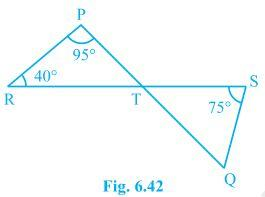Solution:

Consider triangle PRT.

∠PRT +∠RPT + ∠PTR = 180°

So, ∠PTR = 45°

Now ∠PTR will be equal to ∠STQ as they are vertically opposite angles.

So, ∠PTR = ∠STQ = 45°

Again, in triangle STQ,

∠TSQ +∠PTR + ∠SQT = 180°

Solving this we get,

74° + 45° + ∠SQT = 180°

∠SQT = 60°

5. In Fig. 6.43, if PQ ⊥ PS, PQ || SR, ∠SQR = 28° and ∠QRT = 65°, then find the values of x and y.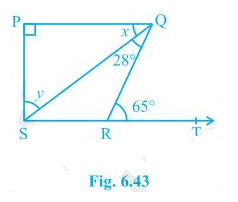Solution:

x +∠SQR = ∠QRT (As they are alternate angles since QR is transversal)

So, x+28° = 65°

∴ x = 37°

It is also known that alternate interior angles are same and so,

∠QSR = x = 37°

Also, Now,

∠QRS +∠QRT = 180° (As they are a Linear pair)

Or, ∠QRS+65° = 180°

So, ∠QRS = 115°

Using the angle sum property in Δ SPQ,

∠SPQ + x + y = 180°

Putting their respective values, we get,

90°+37° + y = 180°

y = 1800 – 1270 = 530

Hence, y = 53°

6. In Fig. 6.44, the side QR of ΔPQR is produced to a point S. If the bisectors of ∠PQR and ∠PRS meet at point T, then prove that ∠QTR = ½ ∠QPR.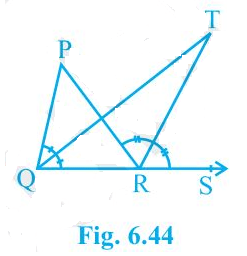Solution:

Consider the ΔPQR. ∠PRS is the exterior angle and ∠QPR and ∠PQR are interior angles.

So, ∠PRS = ∠QPR+∠PQR (According to triangle property)

Or, ∠PRS -∠PQR = ∠QPR ———–(i)

Now, consider the ΔQRT,

∠TRS = ∠TQR+∠QTR

Or, ∠QTR = ∠TRS-∠TQR

We know that QT and RT bisect ∠PQR and ∠PRS respectively.

So, ∠PRS = 2 ∠TRS and ∠PQR = 2∠TQR

Now, ∠QTR = ½ ∠PRS – ½∠PQR

Or, ∠QTR = ½ (∠PRS -∠PQR)

From (i) we know that ∠PRS -∠PQR = ∠QPR

So, ∠QTR = ½ ∠QPR (hence proved).

### NCERT Solutions for Class 9 Maths Chapter 6 – Lines And Angles

The Class 9 Maths theory paper is 80 marks. Out of which Geometry constitutes a total of 22 marks which includes Introduction to Euclid’s Geometry, Lines and Angles, Triangles, Quadrilaterals, Areas, Circles, Constructions. As you can see that it constitutes approximately 27% of weightage. So, using the NCERT Solutions for Class 9, students can easily score high marks by having a thorough understanding of this topic.

Solve all the exercise problems of Lines and Angles. Refer to the NCERT Solutions of Class 9 provided by our Experts below. It will help you to solve the questions in an easy way.

### Summary of NCERT Solutions for Class 9 Maths Chapter 6 Lines And Angles

Before starting to solve the exercise problems, you must first read the theory part and get to know the basic terms, definitions and theorems. After that go through the solved examples of Lines and Angles that are given in the Class 9 NCERT Book. It will make your concepts more clear. Then you can start solving the exercise problems with the help of NCERT Solutions.

### Key Points of NCERT Class 9 Maths Chapter 6 – Lines And Angles

After solving the Line and Angles chapter of Class 9 Maths, you will get to know the following points:

1. Basic terms and definitions related to a line segment, ray, collinear points, non-collinear points, intersecting and non-intersecting lines.
2. Pair of angles (reflex, complementary, supplementary, adjacent, vertical opposite, linear pair).
3. Parallel and Transversal Lines and theorems related to them.
4. Angle sum property of a triangle.

Disclaimer:

Dropped Topics – 6.5 Parallel lines and a transversal and 6.7 Angle sum property of a triangle.